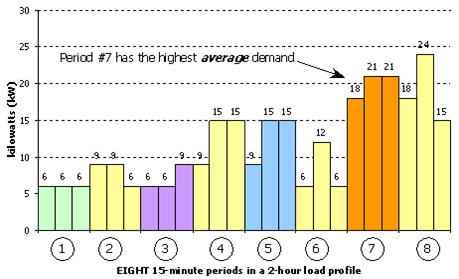### What is Demand?

Your electric bill consists of two components: demand and energy consumption. Demand, or electric load, is measured in kilowatts (kW). Consumption is measured in kilowatt-hours (kWh). Your electric bill is based on both kilowatts and kilowatt hours. The consumption component is based on the total kWh consumed during a given period, regardless of how and when the electricity was used. The demand component of the bill is based on the highest 15 minute demand measured by the electric meter in this period.

But what is demand?

• Demand is the average rate of energy consumption over a 15 minute period.
• Demand measures how fast energy is being consumed (on average) in a 15 minute interval.
• Demand is an average number of electrical loads that run throughout each 15 minute period.

Our demand meters measure a demand for every 15 minute period in each month. The meters record the highest 15 minute demand for the month and retain this figure until the meter is reset by our meter reading equipment. Each meter makes over 2,800 readings per month. Remember, we base your bill on the highest 15 minute reading; therefore, whether it occurs only once in the month or 2,800 times, we charge the same billing demand.

For example, if your business has forty 150 watt light bulbs in hallways that ran continuously, their contribution to demand would be: 40 lamps X 150 watts = 6,000 watts = 6 kW. They would contribute 6 kW to the demand component of your bill. The following example will help to explain demand graphically.

The following example will help to explain demand graphically:The following calculation shows how to calculate the peak demand over the above 2-hour profile:

Period #1: [(6 kW x 5 minutes) + (6 kW x 5 minutes) + (6 kW x 5 minutes)] ¸ 15 minutes = 6.0 kW

Period #2: [(9 kW x 5 minutes) + (9 kW x 5 minutes) + (6 kW x 5 minutes)] ¸ 15 minutes = 8.0 kW

Period #3: [(6 kW x 5 minutes) + (6 kW x 5 minutes) + (9 kW x 5 minutes] ¸ 15 minutes = 7.0 kW

Period #4: [(9 kW x 5 minutes) + (15 kW x 5 minutes) + (15 kW x 5 minutes)] ¸ 15 minutes = 13.0 kW

Period #5: [(9 kW x 5 minutes) + (15 kW x 5 minutes) + (15 kW x 5 minutes)] ¸ 15 minutes = 13.0 kW

Period #6: [(6 kW x 5 minutes) + (12 kW x 5 minutes) + (6 kW x 5 minutes)] ¸ 15 minutes = 8.0 kW

Period #7: [(18 kW x 5 minutes) + (21 kW x 5 minutes) + (21 kW x 5 minutes)] ¸ 15 minutes = 20.0 kW

Period #8: [(18 kW x 5 minutes) + (24 kW x 5 minutes) + (15 kW x 5 minutes)] ¸ 15 minutes = 19.0 kW

At the end of this 2-hour period, the peak demand is 20.0 kW. In a similar way, we perform this analysis over your entire 30-day billing period to determine the billing demand.

### Why do I Get Billed for Demand?

Because electricity cannot be stored in large quantities, the generation, transmission and distribution of electricity needs to have the capacity at all times to meet the demand of all our customers at any given moment. The demand charge on your bill reflects the cost of maintaining the level of service to meet the demand you need, when you need it.

Planning for peak demand is not unique to the electric utility industry. Many businesses address the issue of demand. For example, when designing a highway for public use, it is not enough to know how many vehicles will travel the highway in a day. The designer also must know the maximum number of vehicles that could attempt to use the highway at the same time. This will determine if a two- or four-lane highway is needed to meet the demand.